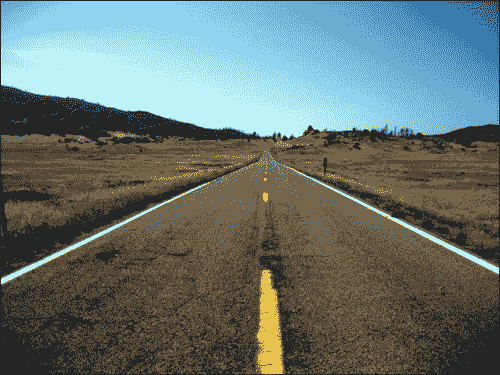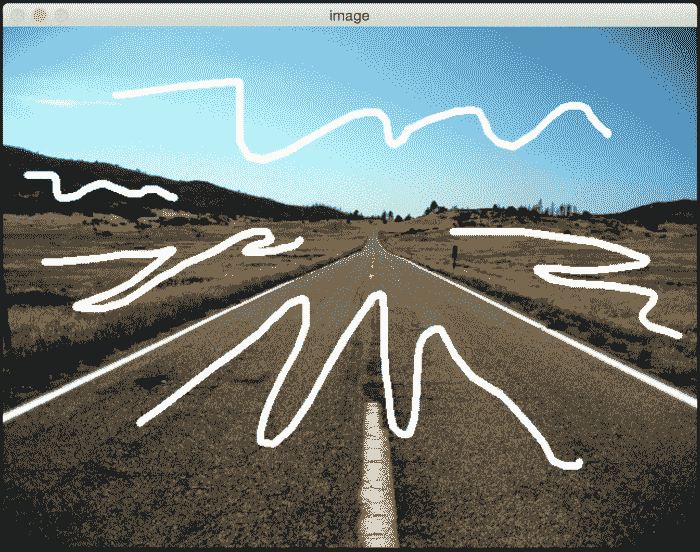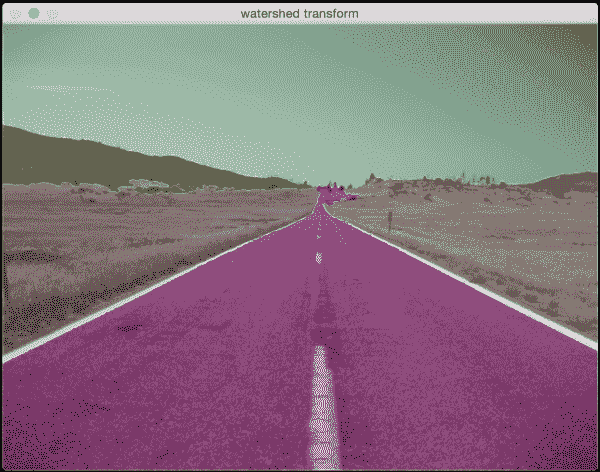# 十二、检测形状和分割图像

• 什么是轮廓分析和形状匹配
• 如何匹配形状
• 什么是图像分割
• 如何将图像分割成其组成部分
• 如何将前景与背景分开
• 如何使用各种技术分割图像

# 轮廓分析和形状匹配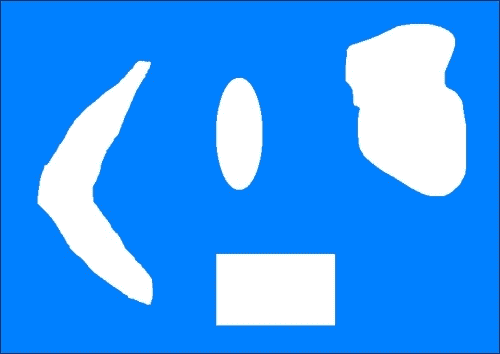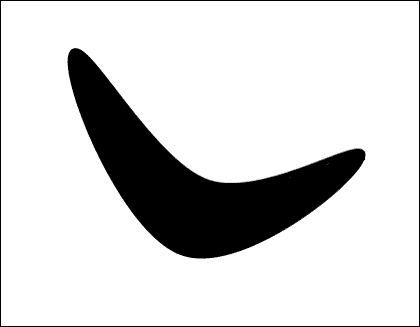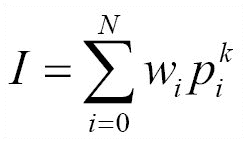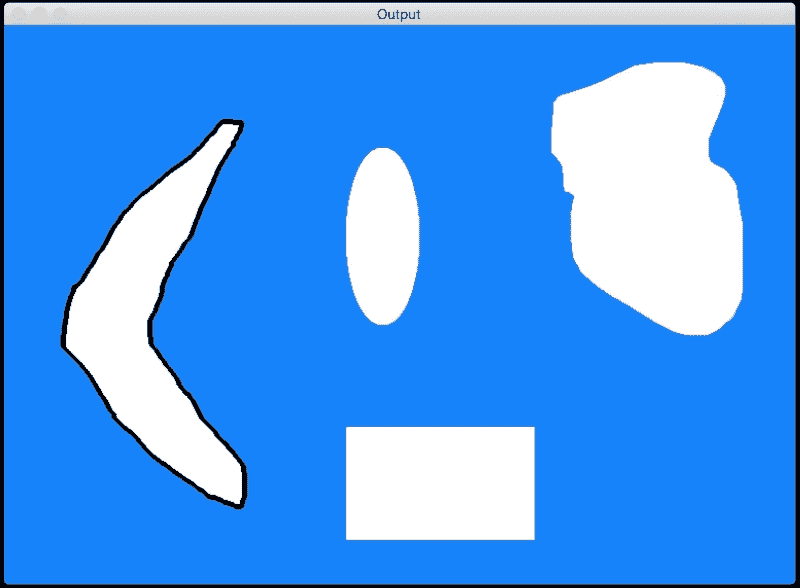import sys

import cv2
import numpy as np

# Extract reference contour from the image
def get_ref_contour(img):
ref_gray = cv2.cvtColor(img, cv2.COLOR_BGR2GRAY)
ret, thresh = cv2.threshold(ref_gray, 127, 255, 0)

# find all the contours in the thresholded image
# The values for the second and third parameters is restricted to a certain number of possible values. You can learn more 'findContours' function here: http://docs.opencv.org/modules/imgproc/doc/structural_analysis_and_shape_descriptors.html
contours, hierarchy = cv2.findContours(thresh, 1, 2)

# Extract the relevant contour based on area ratio
# We use the area ratio because sometimes the image boundary contour is extracted as well and we don't want that. This area ratio threshold will ensure that we only take the contour inside the image.
for contour in contours:
area = cv2.contourArea(contour)
img_area = img.shape * img.shape
if 0.05 < area/float(img_area) < 0.8:
return contour

# Extract all the contours from the image
def get_all_contours(img):
ref_gray = cv2.cvtColor(img, cv2.COLOR_BGR2GRAY)
ret, thresh = cv2.threshold(ref_gray, 127, 255, 0)
contours, hierarchy = cv2.findContours(thresh, 1, 2)
return contours

if __name__=='__main__':
# boomerang reference image

# input image containing all the different shapes

# Extract the reference contour
ref_contour = get_ref_contour(img1)

# Extract all the contours from the input image
input_contours = get_all_contours(img2)

closest_contour = input_contours
min_dist = sys.maxint

# finding the closest contour
for contour in input_contours:
# Matching the shapes and taking the closest one
ret = cv2.matchShapes(ref_contour, contour, 1, 0.0)
if ret < min_dist:
min_dist = ret
closest_contour = contour

cv2.drawContours(img2, [closest_contour], -1, (0,0,0), 3)
cv2.imshow('Output', img2)
cv2.waitKey()



# 近似轮廓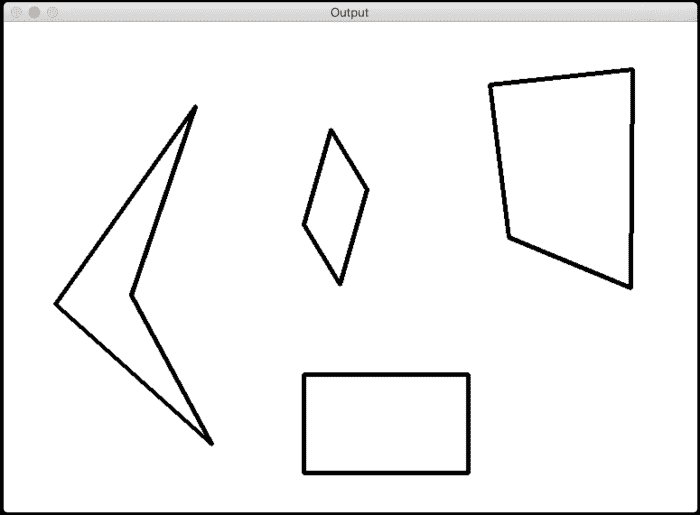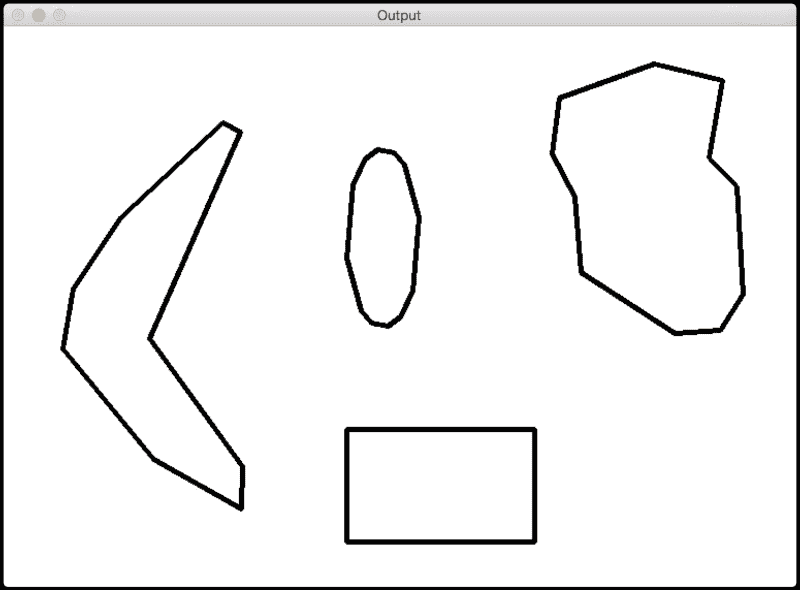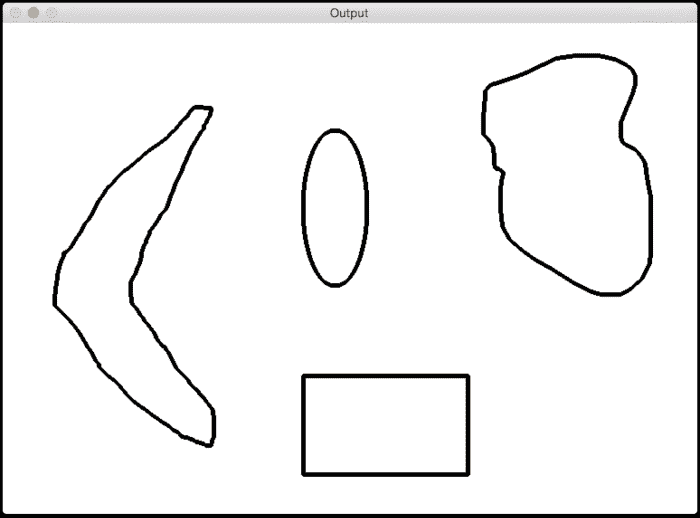# 识别出已切片的披萨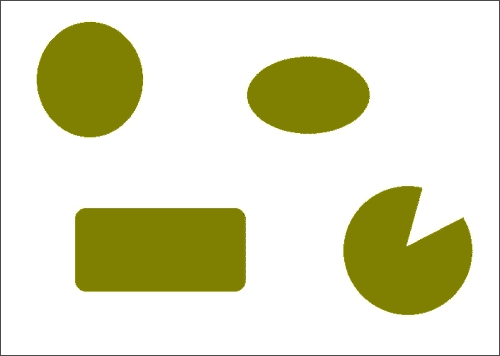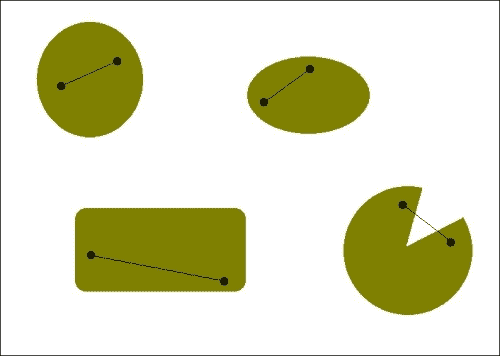import sys

import cv2
import numpy as np

# Input is a color image
def get_contours(img):
# Convert the image to grayscale
img_gray = cv2.cvtColor(img, cv2.COLOR_BGR2GRAY)

# Threshold the input image
ret, thresh = cv2.threshold(img_gray, 127, 255, 0)

# Find the contours in the above image
contours, hierarchy = cv2.findContours(thresh, 2, 1)

return contours

if __name__=='__main__':

# Iterate over the extracted contours
for contour in get_contours(img):
# Extract convex hull from the contour
hull = cv2.convexHull(contour, returnPoints=False)

# Extract convexity defects from the above hull
defects = cv2.convexityDefects(contour, hull)

if defects is None:
continue

# Draw lines and circles to show the defects
for i in range(defects.shape):
start_defect, end_defect, far_defect, _ = defects[i,0]
start = tuple(contour[start_defect])
end = tuple(contour[end_defect])
far = tuple(contour[far_defect])
cv2.circle(img, far, 5, [128,0,0], -1)
cv2.drawContours(img, [contour], -1, (0,0,0), 3)

cv2.imshow('Convexity defects',img)
cv2.waitKey(0)
cv2.destroyAllWindows()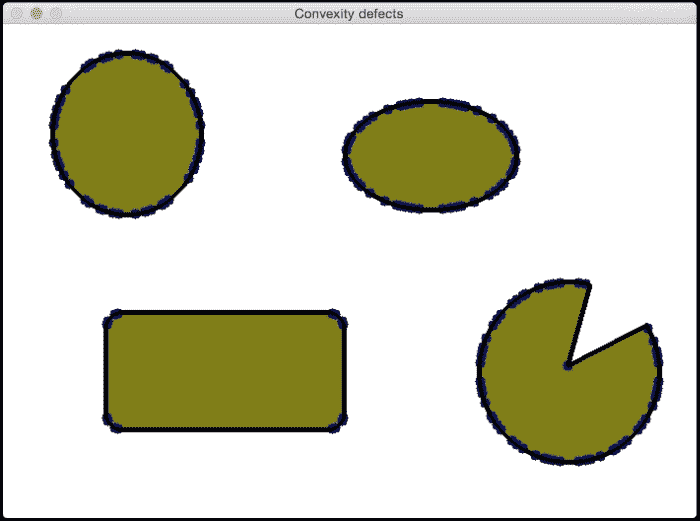import sys

import cv2
import numpy as np

# Input is a color image
def get_contours(img):
img_gray = cv2.cvtColor(img, cv2.COLOR_BGR2GRAY)
ret, thresh = cv2.threshold(img_gray, 127, 255, 0)
contours, hierarchy = cv2.findContours(thresh, 2, 1)
return contours

if __name__=='__main__':

# Iterate over the extracted contours
for contour in get_contours(img):
orig_contour = contour
epsilon = 0.01 * cv2.arcLength(contour, True)
contour = cv2.approxPolyDP(contour, epsilon, True)

# Extract convex hull and the convexity defects
hull = cv2.convexHull(contour, returnPoints=False)
defects = cv2.convexityDefects(contour,hull)

if defects is None:
continue

# Draw lines and circles to show the defects
for i in range(defects.shape):
start_defect, end_defect, far_defect, _ = defects[i,0]
start = tuple(contour[start_defect])
end = tuple(contour[end_defect])
far = tuple(contour[far_defect])
cv2.circle(img, far, 7, [255,0,0], -1)
cv2.drawContours(img, [orig_contour], -1, (0,0,0), 3)

cv2.imshow('Convexity defects',img)
cv2.waitKey(0)
cv2.destroyAllWindows()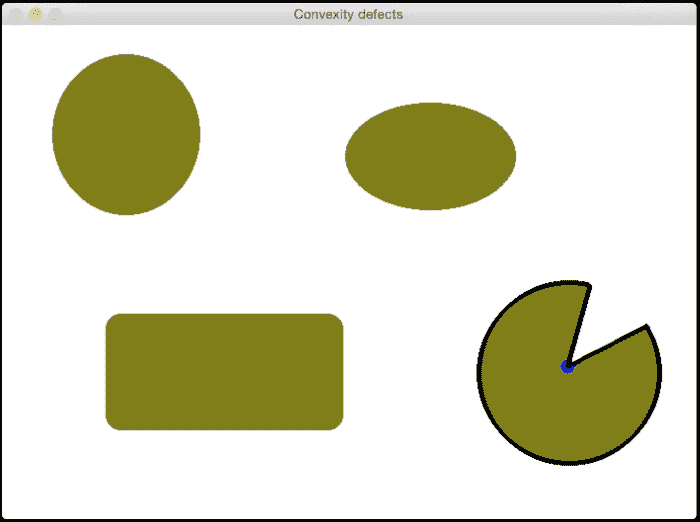# 如何检查形状？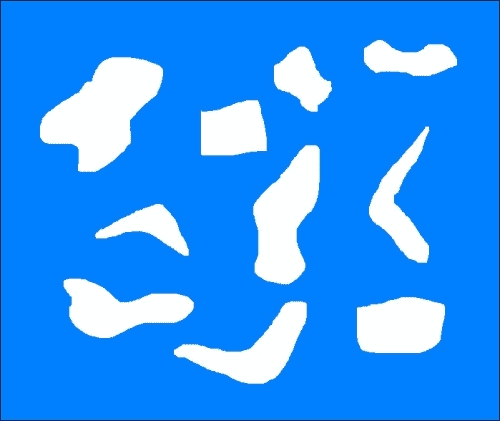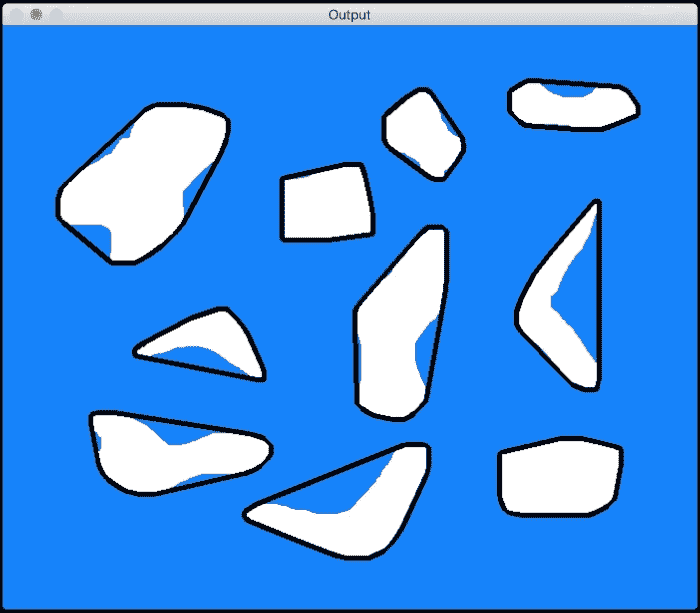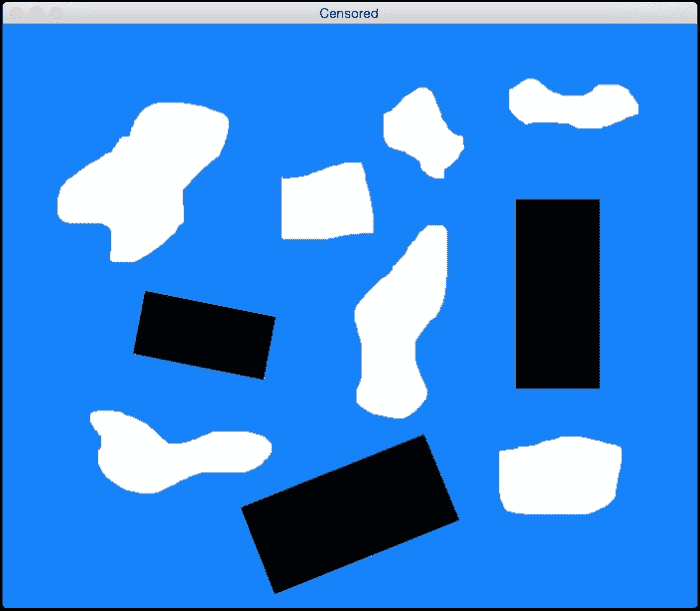import sys

import cv2
import numpy as np

def get_all_contours(img):
ref_gray = cv2.cvtColor(img, cv2.COLOR_BGR2GRAY)
ret, thresh = cv2.threshold(ref_gray, 127, 255, 0)
contours, hierarchy = cv2.findContours(thresh, 1, 2)
return contours

if __name__=='__main__':
# Input image containing all the shapes

img_orig = np.copy(img)
input_contours = get_all_contours(img)
solidity_values = []

# Compute solidity factors of all the contours
for contour in input_contours:
area_contour = cv2.contourArea(contour)
convex_hull = cv2.convexHull(contour)
area_hull = cv2.contourArea(convex_hull)
solidity = float(area_contour)/area_hull
solidity_values.append(solidity)

# Clustering using KMeans
criteria = (cv2.TERM_CRITERIA_EPS + cv2.TERM_CRITERIA_MAX_ITER, 10, 1.0)
flags = cv2.KMEANS_RANDOM_CENTERS
solidity_values = np.array(solidity_values).reshape((len(solidity_values),1)).astype('float32')
compactness, labels, centers = cv2.kmeans(solidity_values, 2, criteria, 10, flags)

closest_class = np.argmin(centers)
output_contours = []
for i in solidity_values[labels==closest_class]:
index = np.where(solidity_values==i)
output_contours.append(input_contours[index])

cv2.drawContours(img, output_contours, -1, (0,0,0), 3)
cv2.imshow('Output', img)

# Censoring
for contour in output_contours:
rect = cv2.minAreaRect(contour)
box = cv2.cv.BoxPoints(rect)
box = np.int0(box)
cv2.drawContours(img_orig,[box],0,(0,0,0),-1)

cv2.imshow('Censored', img_orig)
cv2.waitKey()


# 什么是图像分割？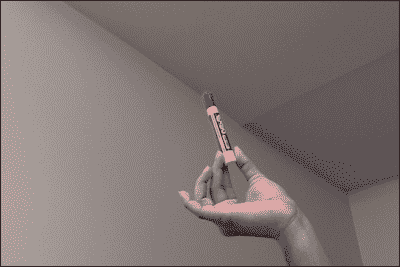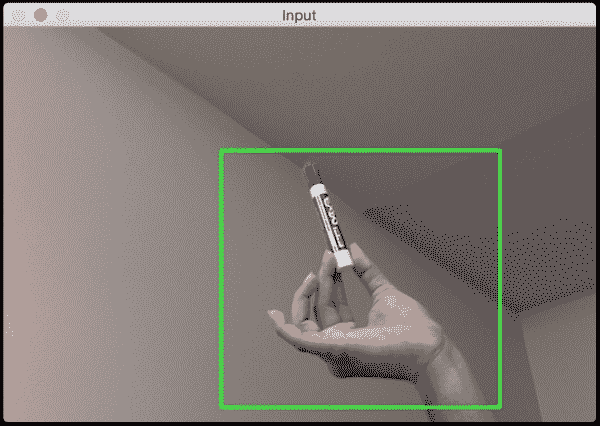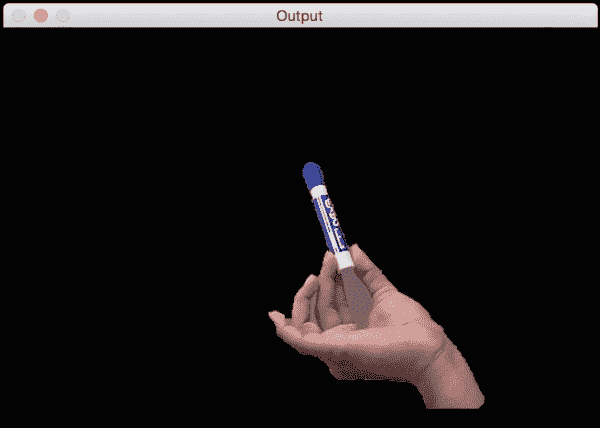import cv2
import numpy as np

# Draw rectangle based on the input selection
def draw_rectangle(event, x, y, flags, params):
global x_init, y_init, drawing, top_left_pt, bottom_right_pt, img_orig

# Detecting mouse button down event
if event == cv2.EVENT_LBUTTONDOWN:
drawing = True
x_init, y_init = x, y

# Detecting mouse movement
elif event == cv2.EVENT_MOUSEMOVE:
if drawing:
top_left_pt, bottom_right_pt = (x_init,y_init), (x,y)
img[y_init:y, x_init:x] = 255 - img_orig[y_init:y, x_init:x]
cv2.rectangle(img, top_left_pt, bottom_right_pt, (0,255,0), 2)

# Detecting mouse button up event
elif event == cv2.EVENT_LBUTTONUP:
drawing = False
top_left_pt, bottom_right_pt = (x_init,y_init), (x,y)
img[y_init:y, x_init:x] = 255 - img[y_init:y, x_init:x]
cv2.rectangle(img, top_left_pt, bottom_right_pt, (0,255,0), 2)
rect_final = (x_init, y_init, x-x_init, y-y_init)

# Run Grabcut on the region of interest
run_grabcut(img_orig, rect_final)

# Grabcut algorithm
def run_grabcut(img_orig, rect_final):

# Extract the rectangle and set the region of
# interest in the above mask
x,y,w,h = rect_final

# Initialize background and foreground models
bgdModel = np.zeros((1,65), np.float64)
fgdModel = np.zeros((1,65), np.float64)

# Run Grabcut algorithm
cv2.grabCut(img_orig, mask, rect_final, bgdModel, fgdModel, 5, cv2.GC_INIT_WITH_RECT)

# Apply the above mask to the image

# Display the image
cv2.imshow('Output', img_orig)

if __name__=='__main__':
drawing = False
top_left_pt, bottom_right_pt = (-1,-1), (-1,-1)

img = img_orig.copy()

cv2.namedWindow('Input')
cv2.setMouseCallback('Input', draw_rectangle)

while True:
cv2.imshow('Input', img)
c = cv2.waitKey(1)
if c == 27:
break

cv2.destroyAllWindows()


# 分水岭算法

OpenCV 附带了分水岭算法的默认实现。 它非常有名，并且有很多实现。 您可以在这个页面中阅读有关的更多信息。 由于您已经可以访问 OpenCV 源代码，因此我们将不在此处查看代码。